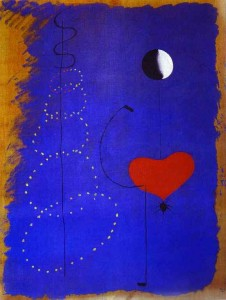# ublobogdan's (micro)blog

## bogdan » surreal numbers

09:15 pm on Sep 10, 2012 | read the article | tags:

the field of surreal numbers is the largest totally ordered field that can be constructed. it’s nice2know because of the elegant construction and the neat ideas behind it.

“given two subsets, L and R of the initial set, with L strictly less than R, {L|R} will denote the number strictly greater than L and strictly less than R”.

we can now elegantly construct:

{|} ~ 0 (where both L and R are empty sets);
{0|} ~ 1 (where L={|} and R is empty);
{1|} ~ 2 … {n|} ~ n+1, thus embedding the natural numbers.

making L empty and R one of the “naturals” we get negative integers: {|n} ~ -n-1

we can extend further the initial set closing it with respect to {0|1} ~ 1/2 and thus embedding the dyadic numbers set, which is a dense set in reals.

following the density of dyadic numbers, for any real number a we can construct infinite dyadic subsets L and R for which L < a < R, i.e. {L|R} ~ a.

the construction goes even further, building transfinite numbers like {{1,2,3,…}|} ~ ω and {0|{1/2,1/4,1/8,…}} ~ ε.

warning:
each surreal number has more than one representation, much like fractions. ex: 1/2 ~ {0|1} ~ {1/4|3/4}. this is why i avoided to use {0|} = 1.

definitions:
field, totally ordered set, dense set, transfinite numberpainting: joan miro – dancer

aceast sait folosește cookie-uri pentru a îmbunătăți experiența ta, ca vizitator. în același scop, acest sait utilizează modulul Facebook pentru integrarea cu rețeaua lor socială. poți accesa aici politica mea de confidențialitate.